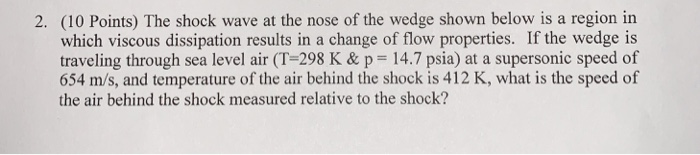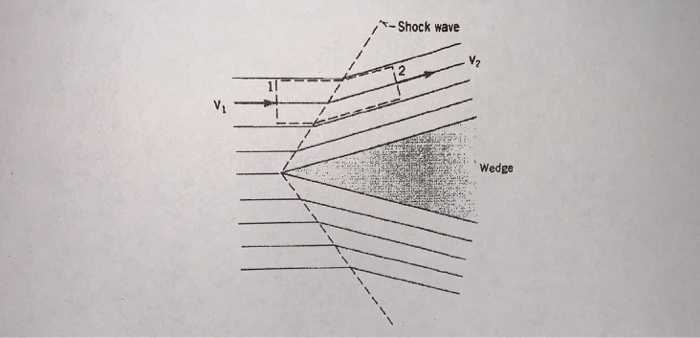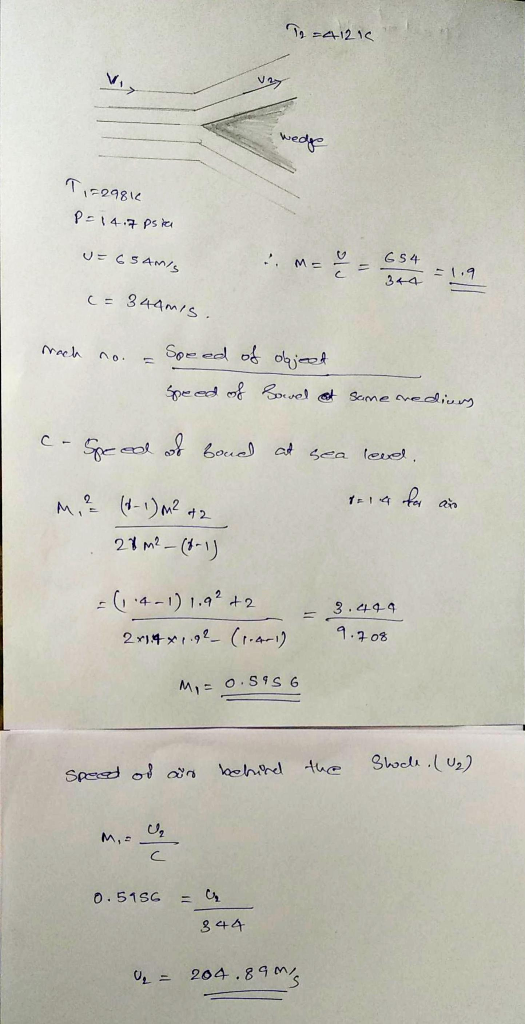Homework Help Question & Answers

2. (10 Points) The shock wave at the nose of the wedge shown below is a region in which viscous d...2. (10 Points) The shock wave at the nose of the wedge shown below is a region in which viscous dissipation results in a change of flow properties. If the wedge is traveling through sea level air (T-298 K & p 14.7 psia) at a supersonic speed of 654 m/s, and temperature of the air behind the shock is 412 K, what is the speed of the air behind the shock measured relative to the shock?
Shock wave V2 WedgeAdd Answer of: 2. (10 Points) The shock wave at the nose of the wedge shown below is a region in which viscous d...
More Homework Help Questions Additional questions in this topic.

• Differential Equation problem A mass of 0.25kg stretches a spring 0.1m. The mass is in a medium that exerts a viscous resistance of 14N when the mass has a velocity of 4m/s. The viscous resistance is...

Need Online Homework Help?

Get FREE EXPERT Answers
WITHIN MINUTES
Related Questions